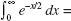# AP Calculus BC Practice Test 23

### Test Information9 questions18 minutes

Calculator Disallowed

1. Find the slope of the curve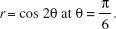2. A particle moves along a line with velocity, in feet per second, v = t2 - t. The total distance, in feet, traveled from t = 0 to t = 2 equals

3. The general solution of the differential equation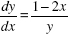is a family of

4. The curve x3 + x tan y = 27 passes through (3,0). Use local linear approximation to estimate the value ofy at x = 3.1. The value is

5.x cos x dx =

6.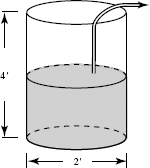The work done in lifting an object is the product of the weight of the object andthe distance it is moved. A cylindrical barrel 2 feet in diameter and 4 feet highis half-full of oil weighing 50 pounds per cubic foot. How much work is done, in foot-pounds,in pumping the oil to the top of the tank?

7. The coefficient of the (x - 8)2 term in the Taylor polynomial for y = x2/3 centered at x = 8 is

8. If f (x) = h(x) and g(x) = x3, then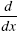f (g(x)) =

9.